## Solve Exercises Lesson 37: Triangular prism and quadrilateral standing prism (Chapter 10 Math 7 Connecting) – Math Book

Solution of Exercises Lesson 37: Triangular vertical prism and quadrilateral standing prism (Chapter 10 Math 7 Connecting)
================

### Solution 10.11 page 98 Math 7 Textbook Connecting knowledge volume 2 – KNTT

Observe and name the bottom, side, bottom, and side faces of the triangular prism in Figure 10.31.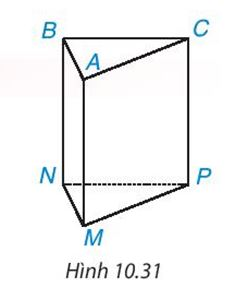Detailed explanation guide

Solution method

– The bottom two sides are parallel to each other.

The side faces are rectangles.

– The sides are parallel and equal.

Detailed explanation

+ 2 bottom faces: ABC, MNP

+ 3 side panels: ACPM, BAMN, BCPN

+ Bottom edge: NM, MP, NP, AB, BC, CA

+ Side: AM, BN, CP

–>

— *****

### Solve problem 10.12 page 99 Math textbook 7 Connecting knowledge volume 2 – KNTT

Observe Figure 10.12 and tell which of the edges (1), (2), (3) joins side AB to have a vertical prism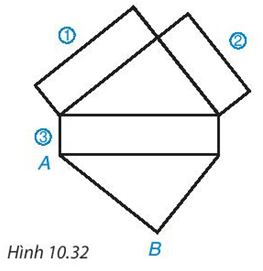Detailed instructions for solving problems 10.12

Solution method

– The bottom two sides are parallel to each other.

The side faces are rectangles.

– The sides are parallel and equal.

Detailed explanation

Side number (1) joins side AB to get a vertical prism.

–>

— *****

### Solve problem 10.13 page 99 Math textbook 7 Connecting knowledge volume 2 – KNTT

Calculate the surrounding area and volume of the vertical prism shown in Figure 10.33.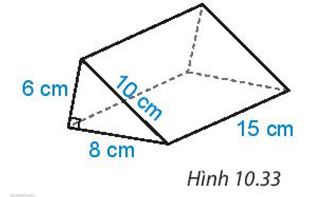Detailed instructions for solving problems 10.13

Solution method

Surrounding area of ​​triangular vertical prism, tetragonal vertical prism:

$${S_{xq}} = Ch$$

where $${S_{xq}}$$: The surrounding area of ​​the prism,

C: Perimeter of one base of the prism,

h: The height of the prism.

Detailed explanation

The area around the vertical prism is:

(6 + 10 + 8) .15 = 360 (m2 )

The area of ​​one base of the prism is:

$\frac{1}{2}$.6.8 = 24 (m2 )

The volume of the vertical prism is

24.15 = 360 ( m3)

–>

— *****

### Solve problem 10.14 page 99 Math textbook 7 Connecting knowledge volume 2 – KNTT

The barrel of an agricultural machine has the shape of a prism standing quadrilateral as shown in Figure 10.34. The bottom of this vertical prism (the side of the barrel) is a square trapezoid with a large base length of 3 m, a small base of 1.5 m. How many cubic meters does the barrel have?Detailed instructions for solving problems 10.14

Solution method

Surrounding area of ​​triangular vertical prism, tetragonal vertical prism:

$${S_{xq}} = Ch$$

where $${S_{xq}}$$: The surrounding area of ​​the prism,

C: Perimeter of one base of the prism,

h: The height of the prism.

Detailed explanation

The area of ​​the base surface of a vertical prism is:

$$\frac{1}{2}$$ (3 + 1.5).1.5 = 3,375 (m .)2)

The volume of a vertical prism is:

3,375.2 = 6.75 (m3)

So the capacity of the barrel will be 6.75 (m3)

–>

— *****

### Solve problem 10.15 page 99 Math textbook 7 Connecting knowledge volume 2 – KNTT

A figure consisting of two vertical prisms combined with the dimensions shown in Figure 10.35. Calculate the volume of the puzzle.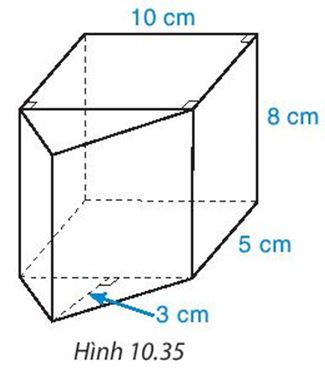Detailed instructions for solving problems 10.15

Solution method

Volumes of a triangular prism and a tetragonal vertical prism:

$$V = {S_{day}}.h$$

where V is the volume of the vertical prism,

Base: Area of ​​one base of a vertical prism,

h: Height of the vertical prism.

Detailed explanation

The volume of a triangular prism is:

$$\frac{1}{2}.3.5.8$$ = 60 (cm3)

The volume of a rectangular prism is:

10. 5. 8 = 400 (cm3)

The volume of the jigsaw is:

60 + 400 = 460 (cm3)

–>

— *****

### Solve problem 10.16 page 99 Math textbook 7 Connecting knowledge volume 2 – KNTT

A medical mask box is made of cardboard in the shape of a rectangular box, the size is as shown in Figure 10.36.

a) Calculate the volume of the box.

b) Calculate the area of ​​cardboard used to make the box (ignoring the gluing edge).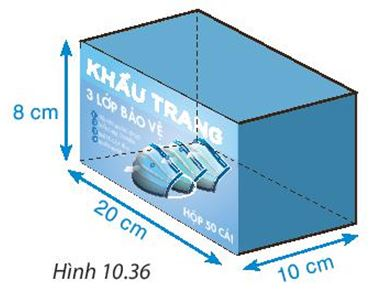Detailed instructions for solving problems 10.16

Solution method

Area of ​​rectangle $${S_{xq}} = 2\left( {a + b} \right).c$$

Detailed explanation

a) The volume of the box is:

20. 10. 8 = 1600 (cm3)

b) The area of ​​cardboard used to make the box is the surrounding area and the area of ​​the two bottoms of the box.

The area around the box is:

2.( 20 + 10 ).8 + 2. 20.10 = 880 (cm3)

–>

— *****